GeeksforGeeks App
Open AppBrowser
Continue

# Class 10 NCERT Solutions- Chapter 11 Constructions – Exercise 11.1

### Question 1. Draw a line segment of length 7.6 cm and divide it in the ratio 5 : 8. Measure the two parts.

Solution:

Steps of construction:

To divide the line segment of 7.6 cm in the ratio of 5 : 8.

Step 1. Draw a line segment AB of length 7.6 cm.

Step 2. Draw a ray AC which forms an acute angle with the line segment AB.

Step 3. Mark the points = 13 as (5+8=13) points, such as A1, A2, A3, A4 …….. A13, on the ray AC such that it becomes AA1 = A1A2 = A2A3 and such like this.

Step 4. Now join the line segment and the ray, BA13.

Step 5. Hence, the point A5, construct a line parallel to BA13 which makes an angle equal to ∠AA13B.

Step 6. Point A5 intersects the line AB at point X.

Step 7. X is that point which divides line segment AB into the ratio of 5:8.

Step 8. Thus, measure the lengths of the line AX and XB. Hence, it measures 2.9 cm and 4.7 cm respectively.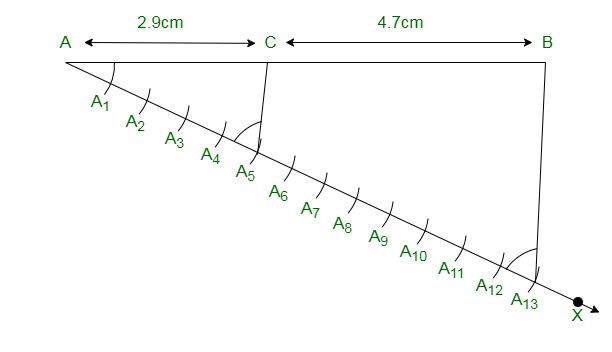Justification:

The construction can be justified by proving thatFrom construction, we have A5X || A13B. By the Basic proportionality theorem for the triangle AA13B, we will get….. (1)

By the figure we have constructed, it can be seen that AA5 and A5A13 contains 5 and 8 equal divisions of line segments respectively.

Thus,… (2)

Comparing the equations (1) and (2), we getThus, Justified.

### Question 2. Construct a triangle of sides 4 cm, 5 cm and 6 cm and then a triangle similar to it whose sides are 2/3 of the corresponding sides of the first triangle.

Solution:

Steps of Construction:

Step 1. Draw a line segment XY which measures 4 cm, So XY = 4 cm.

Step 2. Taking point X as centre, and construct an arc of radius 5 cm.

Step 3. Similarly, from the point Y as centre, and draw an arc of radius 6 cm.

Step 4. Thus, the following arcs drawn will intersect each other at point Z.

Step 5. Now, we have XZ = 5 cm and YZ = 6 cm and therefore ΔXYZ is the required triangle.

Step 6. Draw a ray XA which will make an acute angle along the line segment XY on the opposite side of vertex Z.

Step 7. Mark the 3 points such as X1, X2, X3 (as 3 is greater between 2 and 3) on line XA such that it becomes XX1 = X1X2 = X2X3.

Step 8. Join the point YX3 and construct a line through X2 which is parallel to the line YX3 that intersect XY at point Y’.

Step 9. From the point Y’, construct a line parallel to the line YZ that intersect the line XZ at Z’.

Step 10. Hence, ΔXY’Z’ is the required triangle.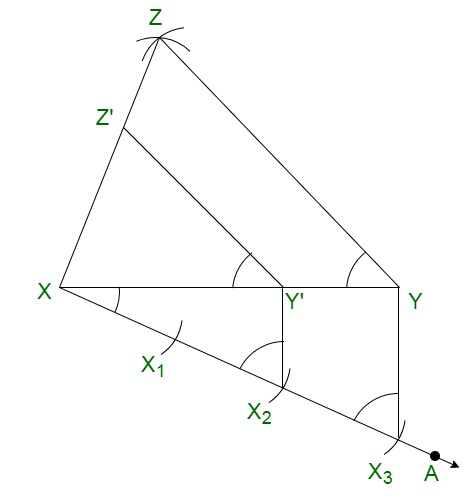Justification:

The construction can be justified by proving thatFrom the construction, we get Y’Z’ || YZ

∴ ∠XY’Z’ = ∠XYZ (Corresponding angles)

In ΔXY’Z’ and ΔXYZ,

∠XYZ = ∠XY’Z (Proved above)

∠YXZ = ∠Y’XZ’ (Common)

∴ ΔXY’Z’ ∼ ΔXYZ (From AA similarity criterion)

Therefore,…. (1)

In ΔXXY’ and ΔXXY,

∠X2XY’ =∠X3XY (Common)

From the corresponding angles, we get,

∠AA2B’ =∠AA3B

Thus, by the AA similarity criterion, we get

ΔXX2Y’ and XX3Y

So,Therefore,……. (2)

From the equations (1) and (2), we obtainIt is written as

XY’ =Y’Z’ =XZ’=Therefore, justified.

### Question 3. Construct a triangle with sides 5 cm, 6 cm, and 7 cm and then another triangle whose sides are 7/5 of the corresponding sides of the first triangle

Solution:

Steps of construction:

Step 1. Construct a line segment XY =5 cm.

Step 2. By taking X and Y as centre, and construct the arcs of radius 6 cm and 5 cm respectively.

Step 3. These two arcs will intersect each other at point Z and hence ΔXYZ is the required triangle with the length of sides as 5 cm, 6 cm, and 7 cm respectively.

Step 4. Construct a ray XA which will make an acute angle with the line segment XY on the opposite side of vertex Z.

Step 5. Pinpoint the 7 points such as X1, X2, X3, X4, X5, X6, X7 (as 7 is greater between 5 and 7), on the line XA such that it becomes XX1 = X1X2 = X2X3 = X3X4 = X4X5 = X5X6 = X6X7

Step 6. Join the points YX5 and construct a line from X7 to YX5 that is parallel to the line YX5 where it intersects the extended line segment XY at point Y’.

Step 7. Now, construct a line from Y’ the extended line segment XZ at Z’ that is parallel to the line YZ, and it intersects to make a triangle.

Step 8. Hence, ΔXY’Z’ is the needed triangle.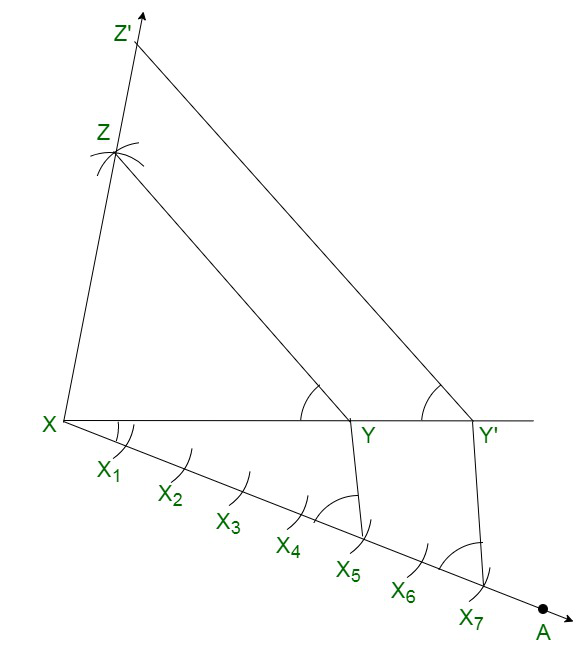Justification:

The construction can be justified by proving that

XY’ =Y’Z’ =XZ’=By the construction, we have Y’Z’ || YZ

Therefore,

∠XY’Z’ = ∠XYZ {Corresponding angles}

In ΔXY’Z’ and ΔXYZ,

∠XYZ = ∠XY’Z   {As shown above}

∠YXZ = ∠Y’XZ’   {Common}

Therefore,

ΔXY’Z’ ∼ ΔXYZ   { By AA similarity criterion}

Therefore,…. (1)

In ΔXX7Y’ and ΔXX5Y,

∠X7XY’=∠X5XY (Common)

From the corresponding angles, we will get,

∠XX7Y’=∠XX5Y

Hence, By the AA similarity criterion, we will get

ΔXX2Y’ and XX3Y

Thus,Hence,……. (2)

From the equations (1) and (2), we obtainIt can be also shown as

XY’ =Y’Z’ =XZ’=Thus, justified.

### Question 4. Construct an isosceles triangle whose base is 8 cm and altitude 4 cm and then another triangle whose sides aretimes the corresponding sides of the isosceles triangle

Solution:

Steps of construction:

Step 1. Construct a line segment YZ of 8 cm.

Step 2. Now construct the perpendicular bisector of the line segment YZ and intersect at the point A.

Step 3. Taking the point A as centre and draw an arc with the radius of 4 cm which intersect the perpendicular bisector at the point X.

Step 4. Join the lines XY and XZ and the triangle is the required triangle.

Step 5. Construct a ray YB which makes an acute angle with the line YZ on the side opposite to the vertex X.

Step 6. Mark the 3 points Y1, Y2 and Y3 on the ray YB such that YY1 = Y1Y2 = Y2Y3

Step 7. Join the points Y2Z and construct a line from Y3 which is parallel to the line Y2Z where it intersects the extended line segment YZ at point Z’.

Step 8. Now, draw a line from Z’ the extended line segment XZ at X’, that is parallel to the line XZ, and it intersects to make a triangle.

Step 9. Hence, ΔX’YZ’ is the required triangle.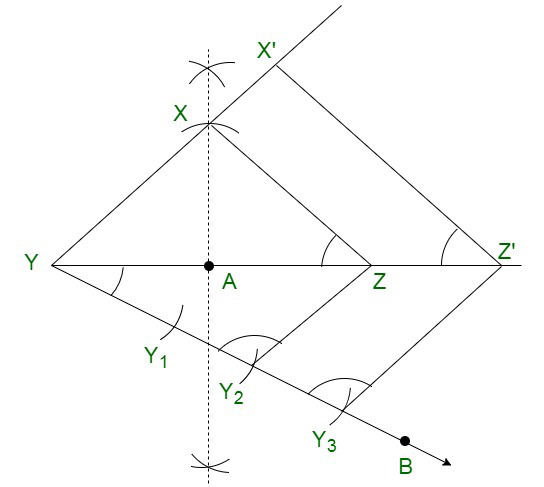Justification:

The construction can be justified by proving that

X’Y =YZ’ =X’Z’=By the construction, we will obtain X’Z’ || XZ

Therefore,

∠ X’Z’Y = ∠XZY {Corresponding angles}

In ΔX’YZ’ and ΔXYZ,

∠Y = ∠Y (common)

∠X’YZ’ = ∠XZY

Therefore,

ΔX’YZ’ ∼ ΔXYZ {By AA similarity criterion}

Hence,Thus, the corresponding sides of the similar triangle are in the same ratio, we getThus, justified.

### Question 5. Draw a triangle ABC with side BC = 6 cm, AB = 5 cm and ∠ABC = 60°. Then construct a triangle whose sides are 3/4 of the corresponding sides of the triangle ABC.

Solution:

Steps of construction:

Step 1. Construct a ΔXYZ with base side YZ = 6 cm, and XY = 5 cm and ∠XYZ = 60°.

Step 2. Construct a ray YA that makes an acute angle with YZ on the opposite side of vertex X.

Step 3. Mark 4 points (as 4 is greater in 3 and 4), such as Y1, Y2, Y3, Y4, on line segment YA.

Step 4. Join the points Y4Z and construct a line through Y3, parallel to Y4Z intersecting the line segment YZ at Z’.

Step 5. Construct a line through Z’ parallel to the line XZ which intersects the line XY at X’.

Step 6. Therefore, ΔX’YZ’ is the required triangle.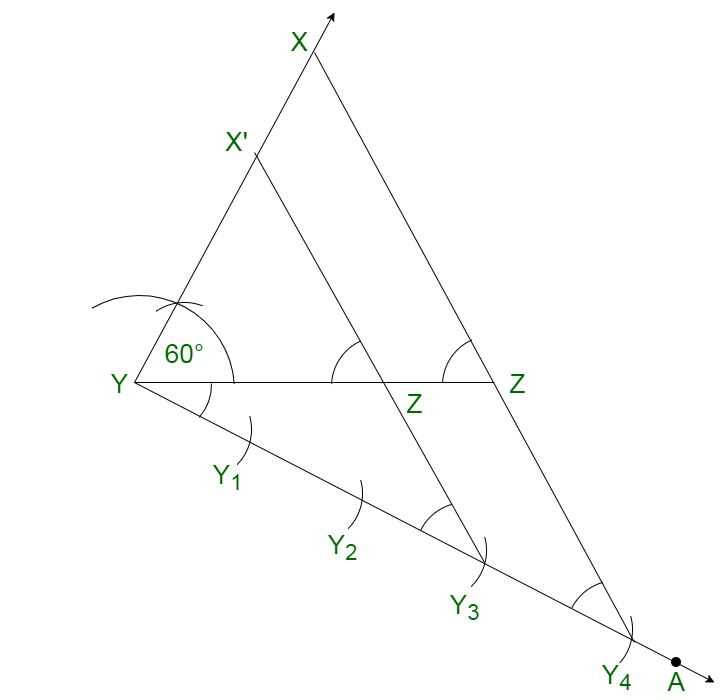Justification:

The construction can be justified by proving that

Since here the scale factor is,

We need to prove

X’Y =YZ’ =X’Z’=From the construction, we will obtain X’Z’ || XZ

In ΔX’YZ’ and ΔXYZ,

Therefore,

∠X’Z’Y = ∠XZY {Corresponding angles}

∠Y = ∠Y {common}

Therefore,

ΔX’YZ’ ∼ ΔXYZ {By AA similarity criterion}

Thus, the corresponding sides of the similar triangle are in the same ratio, we get

Therefore,Thus, it becomesHence, justified.

### Question 6. Draw a triangle ABC with side BC = 7 cm, ∠ B = 45°, ∠ A = 105°. Then, construct a triangle whose sides are 4/3 times the corresponding sides of ∆ ABC.

Solution:

To find ∠Z:

Given:

∠Y = 45°, ∠X = 105°

∠X+∠Y +∠Z = 180° {Sum of all interior angles in a triangle is 180°}

105°+45°+∠Z = 180°

∠Z = 180° − 150°

∠Z = 30°

Thus, from the property of triangle, we get ∠Z = 30°

Steps of construction:

Step 1. Construct a ΔXYZ with side measures of base YZ = 7 cm, ∠Y = 45°, and ∠Z = 30°.

Step 2. Construct a ray YA makes an acute angle with YZ on the opposite side of vertex X.

Step 3. Mark 4 points (as 4 is greater in 4 and 3), such as Y1, Y2, Y3, Y4, on the ray YA.

Step 4. Join the points Y3Z.

Step 5. Construct a line through Y4 parallel to Y3Z which intersects the extended line YZ at Z’.

Step 6. Through Z’, construct a line parallel to the line YZ that intersects the extended line segment at Z’.

Step 7. Hence, ΔX’YZ’ is the required triangle.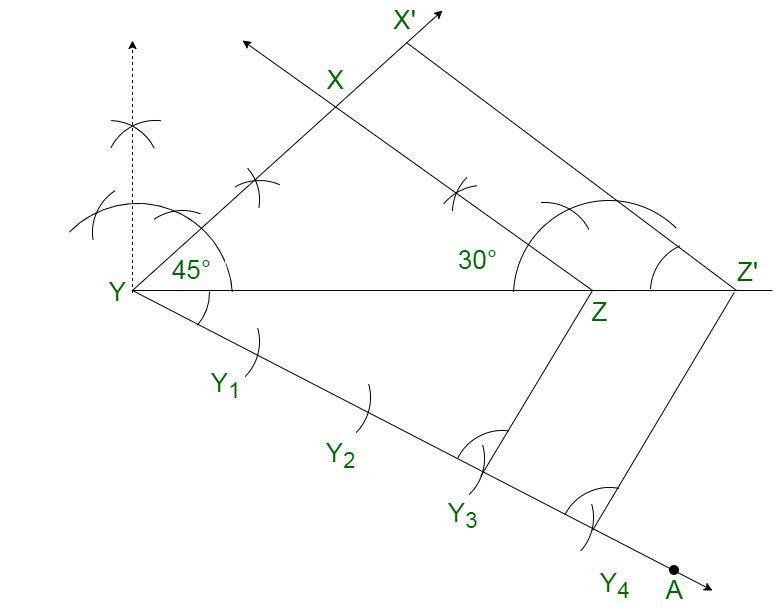Justification:

The construction can be justified by proving that

Here the scale factor is, we have to prove

X’Y =YZ’ =X’Z’=From the construction, we obtain X’Z’ || XZ

In ΔX’YZ’ and ΔXYZ,

Therefore.

∠X’Z’Y = ∠XZY {Corresponding angles}

∠Y = ∠Y {common}

Therefore,

ΔX’YZ’ ∼ ΔXYZ {By AA similarity criterion}

Since the corresponding sides of the similar triangle are in the same ratio, it becomes

Therefore,We get,Thus, justified.

### Question 7. Draw a right triangle in which the sides (other than hypotenuse) are of lengths 4 cm and 3 cm. Then construct another triangle whose sides are 5/3 times the corresponding sides of the given triangle.

Solution:

Given:

The sides other than hypotenuse are of lengths 4cm and 3cm. Hence, the sides are perpendicular to each other.

Step of construction:

Step 1. Construct a line segment YZ =3 cm.

Step 2. Now measure and draw ∠= 90°

Step 3. Now taking Y as centre and draw an arc with the radius of 4 cm and intersects the ray at the point Y.

Step 4. Join the lines XZ and the triangle XYZ is the required triangle.

Step 5. Construct a ray YA makes an acute angle with YZ on the opposite side of vertex X.

Step 6. Mark 5 such as Y1, Y2, Y3, Y4, on the ray YA such that YY1 = Y1Y2 = Y2Y3= Y3Y4 = Y4Y5

Step 7. Join the points Y3Z.

Step 8. Construct a line through Y5 parallel to Y3Z which intersects the extended line YZ at Z’.

Step 9. Through Z’, draw a line parallel to the line XZ that intersects the extended line XY at X’.

Step 10. Therefore, ΔX’YZ’ is the required triangle.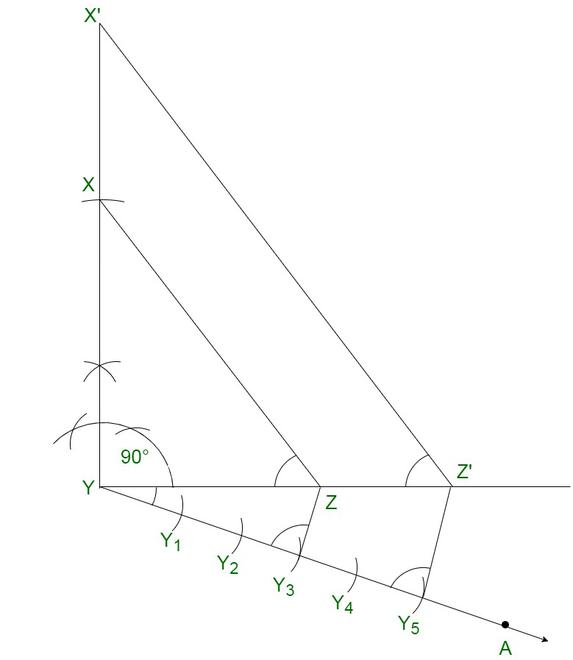Justification:

The construction can be justified by proving that

Here the scale factor is, we need to prove

X’Y =YZ’ =X’Z’=From the construction, we obtain X’Z’ || XZ

In ΔX’YZ’ and ΔXYZ,

Therefore,

∠X’Z’Y = ∠XZY {Corresponding angles}

∠Y = ∠Y {common}

Therefore,

ΔX’YZ’ ∼ ΔXYZ {By AA similarity criterion}

Since the corresponding sides of the similar triangle are in the same ratio, it becomes

Therefore,So, it becomesTherefore, justified.

My Personal Notes arrow_drop_up
Related Tutorials